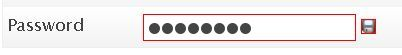## How to read .chm files in Ubuntu

This tutorial assumes that you are a sudoer running Gnome

## What is .chm

Wikipedia says:

Microsoft Compiled HTML Help is a proprietary format for online help files, developed by Microsoft and first released in 1997 as a successor to the Microsoft WinHelp format. It was first introduced with the release of Windows 98, and is still supported and distributed through Windows XP and Vista platforms.

## Install Xchm

The default installation of Ubuntu doesn’t include any reader for CHM help files, but you can add one easily. Continue reading “How to read .chm files in Ubuntu”

The function `generatePassword()` is a simple yet customizable function that generates a random password string, the default password length is 6, you can override it by calling the function like this  `generatePassword(n)` where `n` is the password length. The possible characters are defined within the function with the variable `chars` Continue reading “Generate passwords with JavaScript”

## How to style input fields with CSS?

There are situations where you need to style input fields depending on there types.

Doing this is wrong:

``````input{
border:solid 1px red;
}``````

As you see in the image, the CSS rule has been applied to all input fields Continue reading “How to style input fields with CSS?”

## Maths

Ok, so you already know how to generate positive rondom numbers but let’s do it again:

To generate a random number varying from 0 to 9 we will use this code:

`var randomN = Math.floor(Math.random()*10)`

This expression on the right of the “=”

1. produces a random number from 0 to 1 (excluding 1, say 0.917) with `Math.random()`
2. multiplies it by 10 (9.71)
3. removes decimals (9) with `Math.floor`

For negative values from -9 to 0 we will  simply subtract 9 from the previous result:

`var negativeRandomN = Math.floor(Math.random()*10) - 9`

Now what if you wanted a rondom integer from -10 to 20… ?
Yes you guessed it right! we will generate a random number from 0 to 30 then substact 10 from it

`var randomN = Math.floor(Math.random()*31) - 10`

So the rule is:
To generate a random integer from m to n, here is the code

```var randomN = Math.floor(Math.random() * (n-m+1)) + m
```

## Examples

```# Random integer from 1 to 10
var randomN = Math.floor(Math.random() * 10) + 1

# Random integer from -100 to 100
var randomN = Math.floor(Math.random() * 201) -100

# Random integer from 0 to 255
var randomN = Math.floor(Math.random() * 256)
```

## What is the best free PhotoShop alternative?

The GIMP is the oldest and surely the best free open source image manipulation program, there are many forums that support it an you can find tutorials very easily.## What is email tracking?

E-mail tracking is used by individuals, e-mail marketers, spammers and phishers, to verify that e-mails are actually read by recipients, that email addresses are valid, and that the content of e-mails has made it past spam filters. Continue reading “What is email tracking?”

## On my WordPress theme, How can I find which page is being requested by the visitor?

So you want to know if the visitor is viewing the homepage, a category or a page? WordPress have easy functions for that:

• is_home() : returns true if the visitor is viewing your blog homepage
• is_category() : returns true if viewing a category archive
• is_category(‘3’) : returns true if viewing the archive of the category with the id 3
• is_category(‘People and society’): return true if viewing the archive of the category with the name People and society
• is_category(‘people-society’): return true if viewing the archive of the category with the slug people-society

These are the most used and you can find a lot more on the WordPress Codex.

## How to check if a number is odd or even with PHP?

In mathematics, one of the definitions of odd and even number is that they can be expressed like this (where n is the number and k is an integer):

Odd numbers: n = 2k +1

Even numbers: n = 2k

In PHP, we can check a number’s parity by checking the remainider of the division of this number by 2 Continue reading “How to check if a number is odd or even with PHP?”